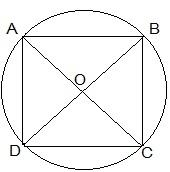Guru

# If diagonals of a cyclic quadrilateral are diameters of the circle through the vertices of the quadrilateral, prove that it is a rectangle. Q.7

• 0

Yesterday i was doing the question from class 9th ncert book of math of Circles chapter of exercise 10.5  What is the easiest way for solving it because i was not able to do this question please help me for solving this question no 7 If diagonals of a cyclic quadrilateral are diameters of the circle through the vertices of the quadrilateral, prove that it is a rectangle.

Share

1. Draw a cyclic quadrilateral ABCD inside a circle with center O such that its diagonal AC and BD are two diameters of the circle.We know that the angles in the semi-circle are equal.

So, ∠ ABC = ∠ BCD = ∠ CDA = ∠ DAB = 90°

So, as each internal angle is 90°, it can be said that the quadrilateral ABCD is a rectangle.

8. If the non-parallel sides of a trapezium are equal, prove that it is cyclic.

• 0